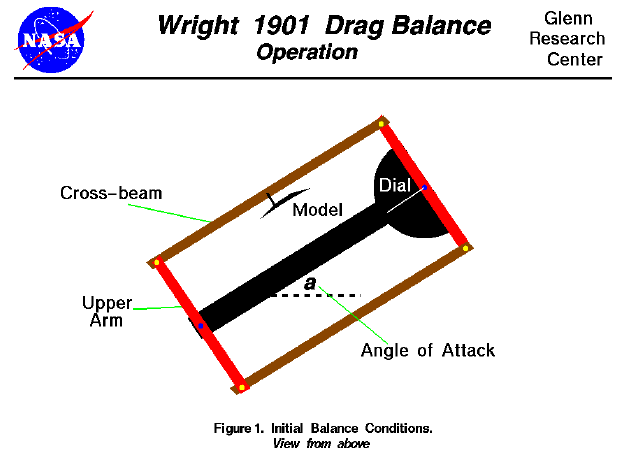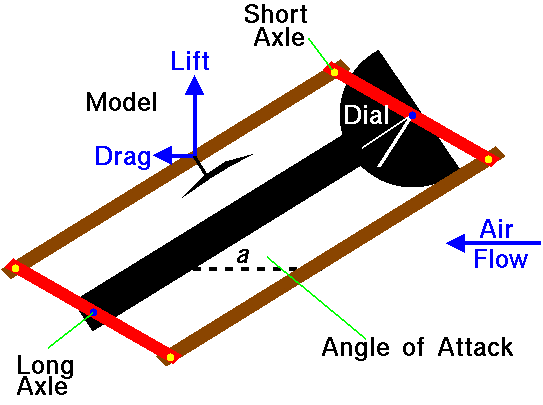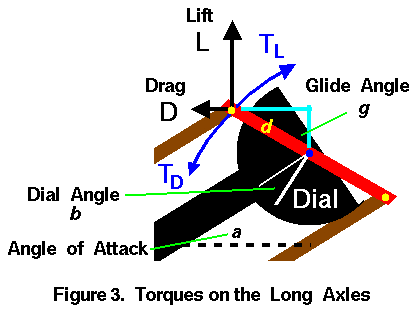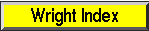+ Text Only Site
+ Non-Flash Version
+ Contact GlennDuring the fall of 1901, the brothers began to question the aerodynamic data on which they were basing their aircraft designs. They decided to conduct a series of wind tunnel tests to verify the results they were experiencing in flight. They would measure the aerodynamic lift and drag on small models of their wing designs using a wind tunnel in their bicycle shop in Dayton, Ohio. They built two separate balances to perform these measurements, one for lift. and the other for drag. On this page we will discuss the operation of the drag balance. On the drag balance, they measured the ratio of the drag of the model to the lift of the model. Then, knowing the lift from the lift balance, they could determine the drag of model. The operation of the balance depends on the concepts of torques and equilibrium. As the test begins, the tunnel is turned off and a model is placed on the cross beam of the balance. The angle of attack of the model is set by rotating the balance on the mounting screw as shown in the figure at the top of this page. The brothers had inscribed lines on the floor of the tunnel to accurately determine the amount of rotation. In this drawing, we are using the same color scheme as used on the page describing the design of the drag balance and we are looking down onto the balance from above. The fan which pushes air through the tunnel is far to the right of this drawing. When the fan is turned on, the cross beams and arms move because of torques generated by the lift and drag force of the model on the long axles. When the torque created by the drag of the model is balanced by the torque generated by the lift of the model, the balance no longer moves and assumes the following orientation:On this figure we show the direction of the forces by the small arrows on the model. The forces generated by the model are transmitted to the arms through the short axles. The arms transmit the force to the long axle and eventually to the frame and tunnel walls. As the arms rotate because of the torque, the pointer of the dial rotates as well. The pointer is always perpendicular to the arm. The torque about a point on an object is equal to the force applied to the object times the perpendicular distance from the point to the place where the force is applied. This figure shows the magnitude and orientation of the torques on the long axles.The length of the arm is given by the symbol d and the arm forms an angle g with a vertical line in the figure. The Wrights called this angle the glide angle. The drag force (D) generates a torque (TD) in the counter-clockwise direction. The distance from the long axle measured perpendicular to the model drag force is d times the cosine of the angle g. The torque (TD) is then given by: TD = D * d * cos(g) The lift force (L) generates a torque (TL) in the clockwise direction. The distance from the long axle measured perpendicular to the model lift force is d times the sine of the angle g. The torque (TL) is then given by: TL = L * d * sin(g) In equilibrium, these torques are equal and the long axle does not rotate. TD = TL D * d * cos(g) = L * d * sin(g) D = L * sin(g) / cos(g) The sine divided by the cosine is equal to the tangent of an angle. Then: D = L * tan(g) We can get mathematical relations for the lift of the model from the lift equation. L= k * V^2 * A * cl where k is the Smeaton coefficient, V is the velocity, A is the surface area of the model, and cl is the lift coefficient. Similarly, the drag equation is: D= k * V^2 * A * cd where cd is the drag coefficient. Substituting into the torque equilibrium equation: k * V^2 * A * cd = k * V^2 * A * cl * tan(g) cd / cl = tan(g) If you compare this equation with the equation for the flight angle of a descending glider, you will see that the angle g is the glide angle experienced in flight. By carefully studying the diagram and using an equation from geometry, we find that the glide angle g is equal to the angle of attack a plus the angle b recorded on the dial. g = a + b The angle b is called the tangential angle. The ratio of the forces along and perpendicular to the cross beam is equal to the tangent of the angle b. Knowing the angle of attack of the model, the Wrights could determine the drag coefficient by recording the angle on the dial, adding the angle of attack, getting the lift coefficient from a previous test and solving the equation given above for cd. They repeated the test process many times, changing models and angle of attack of the models to generate graphs of their results. You can do the same thing, on-line, with an interactive computer simulation of the tunnel and balance which we have developed. Navigation..Beginner's Guide Home Page+ Inspector General Hotline + Equal Employment Opportunity Data Posted Pursuant to the No Fear Act + Budgets, Strategic Plans and Accountability Reports + Freedom of Information Act + The President's Management Agenda + NASA Privacy Statement, Disclaimer, and Accessibility CertificationEditor: Nancy Hall NASA Official: Nancy Hall Last Updated: May 13 2021 + Contact Glenn